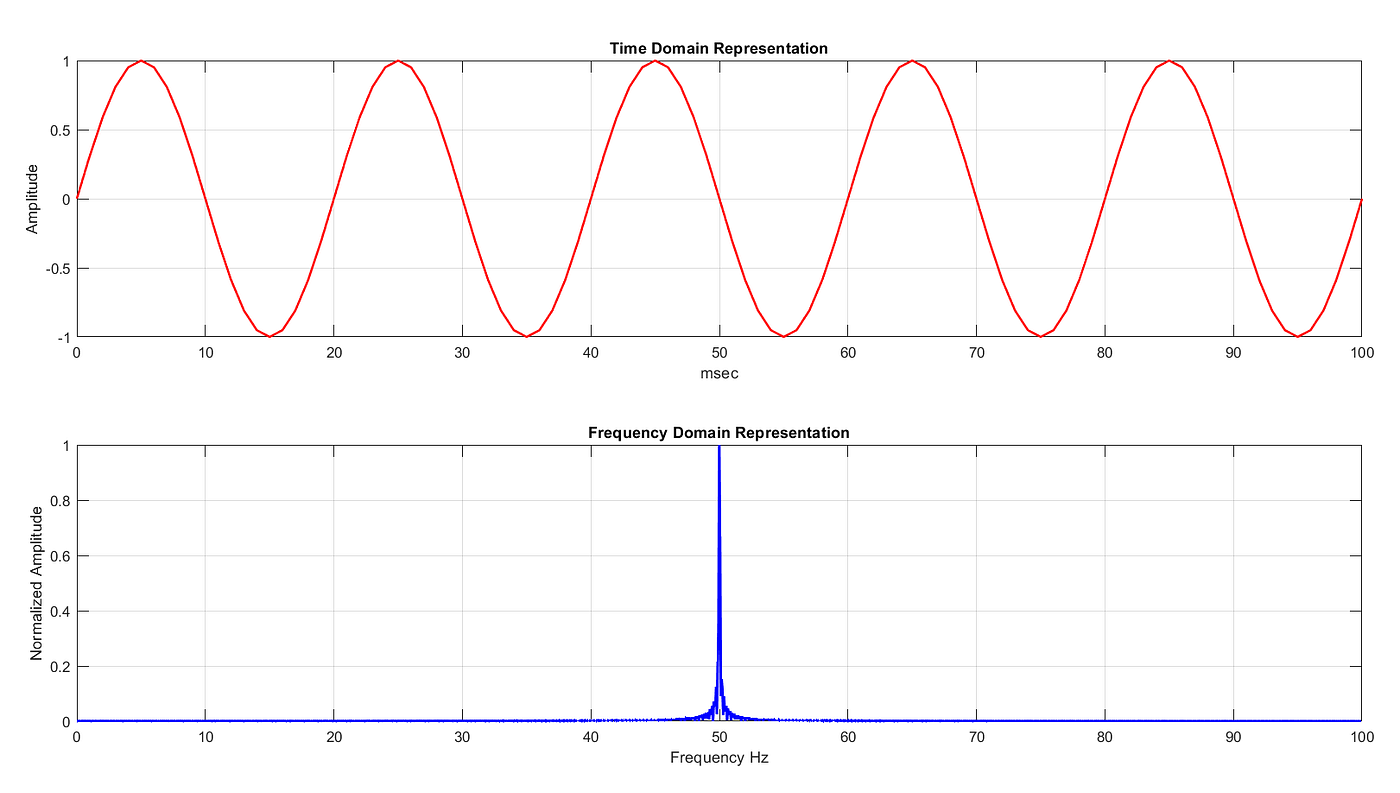# Monte Carlo Simulation : An Astonishing Beauty of Probability

You probably must have studied probability in your secondary schooling, and probably many of you must have got bored with the same subject as it is quite tricky to understand when the problems go beyond coin flip and dice rolling. In practice, there are many situations where finding a probability of something is almost impossible due to variability, uncertainty, and ambiguity. So, how do we find a way from those situations? the answer is to perform Monte Carlo Simulations on your model. Monte Carlo Simulations sometimes called as Monte Carlo Run has a wide range of applications in data science…

# Demystifying the Fourier transform

Fourier transform is the essence of modern data analysis and one of the beauties of mathematics. Fourier transform is a mathematical bridge that joins the time and frequency domain of signal or function representation. If you apply Fourier transform on a function in time domain, you get another function that exists in frequency domain, equivalent to the original time-domain function and vice-versa.

What do time and frequency domains of representation mean?

As shown in the figure below, a sine wave is represented in the both time and frequency domain.Figure 1. Time and frequency domain representation of a signal with a single frequency

In time-domain representation, the signal has variation in amplitude over time…## Aniket kamat

Electronic Engineer, Aerospace Enthusiastic, Amateur Astronomer